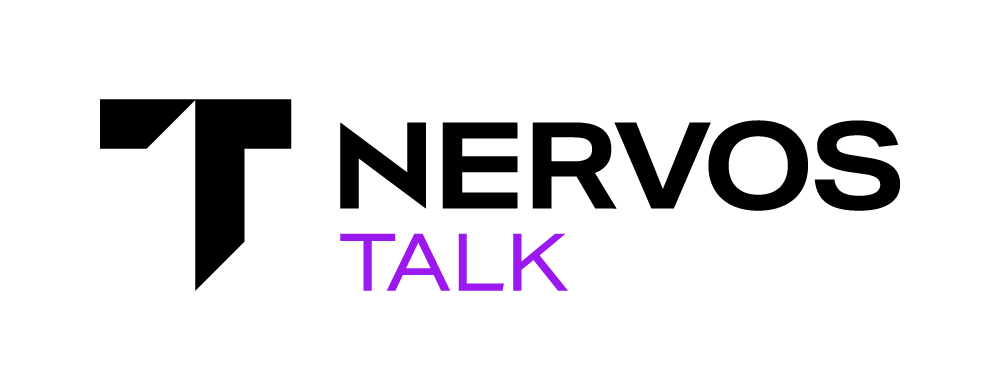# How to parse the DAO field in the CKB block header?

rfcs/0023-dao-deposit-withdraw.md at master · nervosnetwork/rfcs · GitHub says the field encodes 4 integers, how to extract them?

As the chapter Calculation in the RFC23 says, the DAO field packs 4 integers. Each integer is encoded as an unsigned 64-bit little-endian number. That’s 8 bytes for each integer. The DAO field has 32 bytes, so it just concatenates the integers, in this order: `C_i` , `AR_i`, `S_i`, and `U_i`.

For example, in the mainnet, the DAO field of the genesis block is `0x8874337e541ea12e0000c16ff286230029bfa3320800000000710b00c0fefe06`.

We’ll get the serialized integers by splitting it into 4 groups, where each group has 8 bytes. These groups corresponds to `C_i`, `AR_i`, `S_i`, and `U_i` from left to right.

• `C_i`: `0x8874337e541ea12e`
• `AR_i`: `0x0000c16ff2862300`
• `S_i`: `0x29bfa33208000000`
• `U_i`: `0x00710b00c0fefe06`

Then we can decode integers from the binaries, which gives us:

• `C_i`: 3360000145238488200 Shannons, which is about 33.6 billion issued CKB (1 CKB = 10^8 Shannons).
• `AR_i`: 10000000000000000, which is the original value 1 multiplying 10^{16}.
• `S_i`: 35209330473 Shannons
• `U_i`: 504120308900000000 Shannons, which is about 5 billion bytes occupied in the genesis block (1 byte = 1 CKB = 10^8 Shannons).

Following is the example Python code to extract the values:

``````import struct

# The format string `<4q` means:
# < - little endian
# 4 - there are 4 integers
# q - each having 8 bytes
print(struct.unpack('<4q', bytes.fromhex('8874337e541ea12e0000c16ff286230029bfa3320800000000710b00c0fefe06')))

# => (3360000145238488200, 10000000000000000, 35209330473, 504120308900000000)
``````
2 Likes